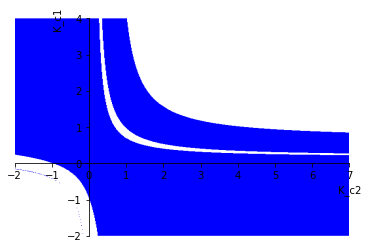# 83. Multivariable Stability analysis¶

When we used proportional control on SISO systems we observed that there is usually an upper bound on the controller gain $$K_c$$ above which the controlled system becomes unstable. Let’s investigate the equivalent calculation for MIMO systems.

:

import sympy
sympy.init_printing()
%matplotlib inline

:

s = sympy.Symbol('s')


This matrix is from example 16.2 in Seborg

:

Gp = sympy.Matrix([[2/(10*s + 1), sympy.Rational('1.5')/(s + 1)],
[sympy.Rational('1.5')/(s + 1), 2/(10*s + 1)]])
Gp

:

$$\left[\begin{matrix}\frac{2}{10 s + 1} & \frac{3}{2 \left(s + 1\right)}\\\frac{3}{2 \left(s + 1\right)} & \frac{2}{10 s + 1}\end{matrix}\right]$$
:

K_c1, K_c2 = sympy.symbols('K_c1, K_c2', real=True)


Unlike in SISO systems, we now have a choice of pairing. We will see that there are differences in the stability behaviour for the different pairings.

:

diagonal = True

:

if diagonal:
Gc = sympy.Matrix([[K_c1, 0],
[0, K_c2]])
else:
Gc = sympy.Matrix([[0, K_c2],
[K_c1, 0]])

:

I = sympy.Matrix([[1, 0],
[0, 1]])


The characteristic equation can be obtained from the $$|I + GpGc|$$. I divide by 4 here to obtain a final constant of 1 like in the example to make comparison easier. Make sure you understand that any constant multiple of the characteristic equation will have the same poles and zeros.

:

charpoly = sympy.poly(sympy.numer((I + Gp*Gc).det().cancel())/4, s)


Compare with Equation 16-20:

:

charpoly2 = sympy.poly(
sympy.numer(
((1 + Gc[0,0]*Gp[0,0])*(1 + Gc[1,1]*Gp[1,1]) - Gc[0,0]*Gc[1,1]*Gp[0,1]*Gp[1,0]).cancel()
)/4, s)

:

charpoly == charpoly2

:

True


Now that we have a characteristic polynomial, we can determine stability criteria using the routh function from tbcontrol.symbolic.

:

from tbcontrol.symbolic import routh

:

R = routh(charpoly)

:

R[0, 0]

:

$$100$$

All the remaining elements of the left hand row must be positive (the same sign as the first element)

:

requirements = True
for r in R[1:, 0]:
requirements = sympy.And(requirements, r>0)


The graph below is supposed to match the textbook, but as of 2019-03-30 it does not. This appears to be a bug in plot_implicit.

:

sympy.plot_implicit(requirements, (K_c2, -2, 7), (K_c1, -2, 4)):

<sympy.plotting.plot.Plot at 0x1217574e0>


As an alternative, let’s evaluate numerically

:

import numpy

:

import matplotlib.pyplot as plt
%matplotlib inline

:

f = sympy.lambdify((K_c2, K_c1), requirements)

:

nK_c2, nK_c1 = numpy.meshgrid(numpy.linspace(-2, 4, 300), numpy.linspace(-2, 7, 300))

:

r = f(nK_c2, nK_c1)

:

plt.pcolor(nK_c2, nK_c1, r)
plt.ylabel('K_c1')
plt.xlabel('K_c2')

:

Text(0.5, 0, 'K_c2')We can see that even this simple system can exhibit more complicated behaviour than we may expect from first order systems because of the extra loops formed by the controllers.